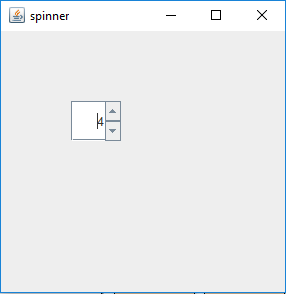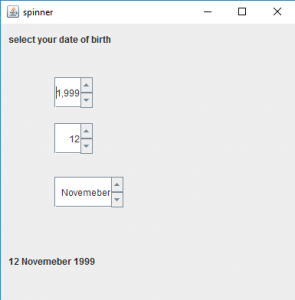GFG App
Open AppBrowser
Continue

JSpinner is a part of javax.swing package. JSpinner contains a single line of input which might be a number or a object from an ordered sequence. The user can manually type in a legal data into the text field of the spinner. The spinner is sometimes preferred because they do not need a drop down list. Spinners contain an upward and a downward arrow to show the previous and the next element when it is pressed.

Constructors of JSpinner are:

1. JSpinner(): Creates an empty spinner with an initial value set to zero and no constraints.
2. JSpinner( SpinnerModel model): Creates a spinner with a specified spinner model passed as an argument.

Commonly used methods are :

1. SpinnerListModel(List l): creates a spinner model with elements of list l. This spinner model can be used to set as a model for spinner.
2. SpinnerNumberModel(int value, int max, int min, int step): returns a spinner model whose initial value is set to value, with minimum and maximum value, and a definite step value.
3. setValue(Object v): sets the value of the spinner to the object passed as argument.
4. getValue(): returns the current value of the spinner.
5. getPreviousValue(): returns the previous value of the spinner.
6. getNextValue(): returns the next value of the spinner.

1. Program to create a simple JSpinner

## Java

 `// java Program to create a` `// simple JSpinner` `import` `java.awt.event.*;` `import` `javax.swing.*;` `import` `java.awt.*;` `class` `spinner ``extends` `JFrame {` `    ``// frame` `    ``static` `JFrame f;`   `    ``// default constructor` `    ``spinner()` `    ``{` `    ``}`   `    ``// main class` `    ``public` `static` `void` `main(String[] args)` `    ``{` `        ``// create  a new frame` `        ``f = ``new` `JFrame(``"spinner"``);`   `        ``// create a JSpinner` `        ``JSpinner s = ``new` `JSpinner();`   `        ``// set Bounds for spinner` `        ``s.setBounds(``70``, ``70``, ``50``, ``40``);`   `        ``// set layout for frame` `        ``f.setLayout(``null``);`   `        ``// add panel to frame` `        ``f.add(s);`   `        ``// set frame size` `        ``f.setSize(``300``, ``300``);`   `        ``f.show();` `    ``}` `}`

Output :2. Program to create a JSpinner and add ChangeListener to it; Program to select your date of birth using JSpinner.

## Java

 `// Java program to select your` `// date of birth using JSpinner` `import` `java.awt.event.*;` `import` `javax.swing.*;` `import` `java.awt.*;` `import` `javax.swing.event.*;` `class` `spinner1 ``extends` `JFrame ``implements` `ChangeListener {` `    ``// frame` `    ``static` `JFrame f;`   `    ``// label` `    ``static` `JLabel l, l1;`   `    ``// spinner` `    ``static` `JSpinner s, s1, s2;`   `    ``// default constructor` `    ``spinner1()` `    ``{` `    ``}`   `    ``// main class` `    ``public` `static` `void` `main(String[] args)` `    ``{` `        ``// create an object of the class` `        ``spinner1 sp1 = ``new` `spinner1();`   `        ``// create  a new frame` `        ``f = ``new` `JFrame(``"spinner"``);`   `        ``// create a label` `        ``l = ``new` `JLabel(``"select your date of birth"``);` `        ``l1 = ``new` `JLabel(``"1 January 2000"``);`   `        ``// create a JSpinner with a minimum, maximum and step value` `        ``s = ``new` `JSpinner();` `        ``s1 = ``new` `JSpinner(``new` `SpinnerNumberModel(``1``, ``1``, ``31``, ``1``));`   `        ``// setvalue of year` `        ``s.setValue(``2000``);`   `        ``// store the months` `        ``String months[] = { ``"January"``, ``"February"``, ``"March"``,` `        ``"April"``, ``"May"``, ``"June"``, ``"July"``, ``"August"``, ` `        ``"September"``, ``"October"``, ``"Novemeber"``, ``"December"` `};`   `        ``// create a JSpinner with list values` `        ``s2 = ``new` `JSpinner(``new` `SpinnerListModel(months));`   `        ``// add change listener to spinner` `        ``s.addChangeListener(sp1);` `        ``s1.addChangeListener(sp1);` `        ``s2.addChangeListener(sp1);`   `        ``// set Bounds for spinner` `        ``s.setBounds(``70``, ``70``, ``50``, ``40``);` `        ``s1.setBounds(``70``, ``130``, ``50``, ``40``);` `        ``s2.setBounds(``70``, ``200``, ``90``, ``40``);`   `        ``// setbounds for label` `        ``l.setBounds(``10``, ``10``, ``150``, ``20``);` `        ``l1.setBounds(``10``, ``300``, ``150``, ``20``);`   `        ``// set layout for frame` `        ``f.setLayout(``null``);`   `        ``// add label` `        ``f.add(l);` `        ``f.add(l1);` `        ``f.add(s);` `        ``f.add(s1);` `        ``f.add(s2);`   `        ``// add panel to frame` `        ``f.add(s);`   `        ``// set frame size` `        ``f.setSize(``400``, ``400``);`   `        ``f.show();` `    ``}`   `    ``// if the state is changed` `    ``public` `void` `stateChanged(ChangeEvent e)` `    ``{` `        ``l1.setText(s1.getValue() + ``" "` `+ s2.getValue() + ``" "` `+ s.getValue());` `    ``}` `}`

Output :Note: This program will not run in an online compiler please use an Offline IDE.

My Personal Notes arrow_drop_up